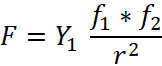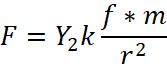# Dark Matter vs MOND vs Gravity by EM Waves

A comparative examination of cosmological observations through three theories

Alexander Z. Yan

Traditional Newtonian physics cannot explain many observed galactic-scale gravitational effects and phenomena. This has led to many new theories, but most cannot explain all phenomena simultaneously. This is shown in Table 1.

One such theory is Dark Matter. The current theory suggesting that Dark Matter is comprised of tiny particles is generally accepted; however, this theory faces some tough challenges such as the Tully-Fisher Relation and the Core-Cusp Problem. To date, no such particle has been detected after more than three decades of research.

Due to the obvious problems with the above Dark Matter theory, some alternatives have been proposed. One of them is the MOND (Modified Newtonian dynamics) theory, which can explain some observations neatly, but this theory is also struggling with some other observations, such as the Bullet Cluster, Gravitational Lensing, CMB, and expansion of the universe.

In 2020, a new unified field theory on gravity was published that can explain all the above phenomenon [1,2]. The new field theory postulates that if gravity forces are due to an elastic field, it would also be exerted by electromagnetic waves or photons of light. According to this new theory, this is where the extra gravity force on those so-called Dark Matter observations comes from. The equations for the new force are as follows:(Gravity force between photon and photon)(Gravity force between photon and object with mass)

Where:

F – gravity force between two photons or between a photon and an object with mass (N)
Y1 = 3.61 × 10−111
𝑚3 𝑘g
Y2 = 4.91 × 10-61 m3 s-1
k – number of photons of the same frequency
f – photon frequency (Hz)
m – mass of object (kg)
r – distance between object with mass and photon (m)

Table 1– Comparison of Different Theories on Phenomenon Each can Explain

 Dark Matter MOND Gravity by EM waves Galaxy Rotation Curve Yes Yes Yes Gravitational Lensing Yes No Yes Bullet Cluster Yes No Yes Tully-Fisher Relation No Yes Yes Dark Matter Halo No No Yes Core-Cusp problem No Yes Yes Data on Cosmological scale Yes No Yes

The following is a brief comparison of the Dark Matter Particle, MOND, and Gravity from EM Waves theories using the cosmological observations and phenomena in Table 1.

- Galaxy Rotation Curve

A galaxy’s rotation curve is a plot of the orbital speeds of a star or gas as a function of the radial distance from the center of the galaxy. A discrepancy appears when comparing observed and expected rotation curves when applying classical Newtonian equations.

All three theories can explain this discrepancy.

- Gravitational Lensing

Gravitational lensing occurs when light bends due to a force from a distribution of matter. Dark Matter can explain gravitational lensing, but MOND has difficulty explaining this observation.

Gravity by EM waves can explain gravitational lensing. The additional gravitational force exerted by EM waves emitted from a galaxy would lead to an increase in gravitational force observed as gravitational lensing.

- Bullet Cluster

The Bullet Cluster (1E 0657-56) consists of two colliding clusters of galaxies. During the collision, gravitational lensing showed that the gravity field of the colliding galaxies maintained a velocity after the galaxies themselves slowed down.

Dark Matter claims this as the best evidence for the existence of dark matter, but it cannot explain why the gravitational field does not slow down. This implies dark matter as tiny particles does not collide or experience friction, something that the Dark Matter particle theory cannot explain.

MOND cannot explain the Bullet Cluster phenomenon at all.

Gravity by EM waves can explain the Bullet Cluster observations. EM waves emitted by each colliding galaxy continue to surround that galaxy and maintain momentum even after the galaxies slow down. EM waves, instead of particles, don’t interact at all with each other but pass right through.

- Tully-Fisher Relation

The Tully-Fisher Relation is an empirical relationship between the asymptotic rotational velocity at the edge of a spiral galaxy and the galaxy’s luminosity. This solid observation makes no sense under the theory of Dark Matter as a tiny particle. This is a major reason why the theory of Dark Matter is being seriously questioned by the scientific community .

MOND can explain the Tully-Fisher Relation well.

The theory of Gravity by EM waves can also easily explain the Tully-Fisher Relation. According to the gravity force by EM waves equations, the photon’s frequency and number are both variables that affect the gravity force. The higher the frequency and the greater the number of photons, the stronger the gravity force will be. This allows for a faster rotation speed of the objects at the edge of the galaxy. Hence there is correlation between luminosity of a galaxy and its velocity of rotation. The Tully-Fisher relation matches the Gravity by EM Waves theory.

- Dark Matter Halo

Although galaxies are typically flat, galaxies have a roughly spherical gravitational field. Dark Matter proponents suggest that this is due to dark matter, but no explanation can be made as to how.

MOND also cannot provide an explanation for this observation.

Gravity by EM waves can explain this. Galaxies emit EM waves in all directions, producing the spherical gravitational field. As EM waves move further out, their energy dissipates and thereby having a weaker gravitational effect further away. This results in the observed Dark Matter halos.

- Core-Cusp Problem

The Core‐Cusp Problem refers to a discrepancy between inferred dark matter and simulated density profiles of low-mass galaxies. Poses a problem for current Dark Matter theories, and there remains a discrepancy between theory and observation regarding the mass‐density distribution of the dark matter halo.

MOND can explain the Core-Cusp Problem.

The Gravity by EM wave theory won’t contradict the observations of how Dark Matter behaves because EM waves are emitted and spread out in all directions from a galaxy.

- Observations on how the universe formed and its current structure, such as Cosmic Microwave Background (CMB) and Lambda Cold Dark Matter (ΛCDM)

The CMB and ΛCDM observations support Dark Matter theory.

Our current understanding of the universe and CMB and ΛCDM observations challenge MOND. This is one of the major problems with MOND.

Gravity by EM waves not only explains the above observations, but it also offers new solutions to those problems. Constants, density, smoothness, accelerated rate of expansion of our universe, dark energy, etc. can be answered with the Gravity by EM Waves theory.

The comparison above shows that neither Dark Matter nor MOND theories can explain all the cosmological observations. Attempts to combine these theories have complicated the study. However, the Gravity by EM Waves theory can explain these cosmological observations. As the scientific community often follows Occam’s razor, this new unified field theory deserves serious examination.

References

 Yan, Max Gaofei. (2021). “Equations of force between photons or photon and matter with mass,” Zenodo. https://doi.org/10.5281/zenodo.4541280

 Yan, Max Gaofei. (2020). “Unified Field Theory for Gravity and Electromagnetism and Its Explanation for Dark Matter Observations,” Zenodo. https://doi.org/10.5281/zenodo.4057270

 Lincoln, D. (2017, July 16). Is dark matter real? LiveScience. From https://www.livescience.com/59814-is-dark-matter-real.html

Article Sort
1
Article Section